# Chemistry 3 Flashcards Preview

## ALTIUS > Chemistry 3 > Flashcards

Flashcards in Chemistry 3 Deck (68):
1

1

2

## Solution Chemistry Ways of measuring & describing solution [ ] Compare Molarity with Molality How do they both change with temperature?

### Molarity, M =moles solute/Liter solution Molality, m =moles solute/Kg solvent ​ Molarity (M) changes w/ temperature, but molality (m) DOES NOT! Provide a possible explanation... Hint: what happens to volume when temperature increases? (increases. Mass doesnt change though)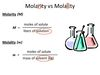3

3

## Solution Chemistry Ions in Solution What do Na+ and Cl- look like in water? In specific, how are the dipoles oriented?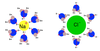4

5

7

7

7

8

8

9

11

## Electrochemical cells Galvanic Cells What does the salt bridge do?

### There will be a buildup of negative charge in the copper vessel due to continual loss of copper cations and a buildup of positive charge in the zinc vessel due to the continual production of zinc cations. This polarity resists the flow of electrons and would eventually shut down the cell if a salt bridge were not present. Within the salt bridge sodium ions can flow toward the copper vessel and nitrate ions can flow toward the zinc vessel, neutralizing the buildup of charge and allowing electron flow to continue. The metal cations themselves, as well as any other ions in the solutions, can also flow through the salt bridge. In an electrical sense, the salt bridge connects the circuit, allowing continual flow of electrons from electrode to electrode and then back through the salt bridge via ion diffusion.11

## Phase changes Heating Curves  Describe them

### A graph of temperature (T) in Kelvin or Celsius vs. heat (q) in Joules. Occasionally, time is graphed on the x-axis instead of heat (if heat is added at a constant rate the temperature vs. heat graph and the temperature vs. time graph look approximately the same).12

## Phase Changes Describe Osmotic Pressure What side RECEIVES water?  More___=More OSMOTIC PRESSURE

### A measure of the tendency of water to move from one solution to another across a semi-permeable membrane Usually represented by the capital Greek letter pi, Π. It is the side that will receive the water via osmosis that has the higher osmotic pressure. In other words, more solute means more osmotic pressure. Π= iMRT i = # of ions formed in solution M is the solute molarity R is the gas constant T is the absolute temperature13

14

15

15

16

17

## Gases Dalton's Law of Partial Pressures

### Ptotal=P1+P2+P3.... This seems pretty straightforward; the sum of the partial pressures equals the total pressure. However, if you gloss over this you will miss a very important point. If we add more of Gas 1 (P1) to an existing mixture of three gases, we have increased the partial pressure of Gas 1 and the total pressure, but have had zero effect on the partial pressure of the other gases. Partial pressure is NOT similar to mole fraction or mass percent. By adding more of Gas 1 we did decrease the mole fraction and the mass fraction of Gases 2 and 3, but we did NOT decrease their partial pressures.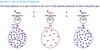18

## Phase Changes Heating Curves What do horizontal portions of the graph represent? What does the slope of the various non-horizontal sections of the graph represent?

### The horizontal sections of the graph represent phase changes. The first flat section will represent the phase change between solid and liquid and the second will represent the phase change between liquid and gas. If heat is on the x-axis then the length of the first horizontal section represents the heat of fusion and the length of the second horizontal section represents the heat of vaporization. The slope of the lines between these horizontal sections represents the inverse (∆T/Q) of heat capacity (Q/∆T) for that particular phase of the substance. One should observe, therefore, that different phases of the same substance usually have different heat capacities—as indicated by the differing slopes of those sections of the following graph:18

## Phase Changes Vapor Pressure Raoult's Law What does Raoult's Law solve for? Give the formula for vapor pressure w/ a NON-Volatile solute

### SOLVES FOR VAPOR PRESSURE OF A SOLVENT THAT HAS A SOLUTE ADDED TO ITVpTotal = XVp°  Vapor Pressure w/ a Non-Volatile Solute =(mole fraction of the pure solvent, X)X (Vp of the pure solvent, Vp°)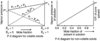19

## Phase Changes Draw a phase diagram. Label: axes solid, liquid,  gas regions critical point triple point Describe what's going on at the critical & triple points wrt phases

### The triple point  the precise temperature and pressure at which all three phases (i.e., states) exist simultaneously in equilibrium with each other. The critical point  the precise temperature and pressure above which liquid and gas phases become indistinguishable. At this point liquid and gas phases cease to exist, merging into a single phase called a supercritical fluid. This supercritical fluid cannot be compressed back into the liquid phase by increasing pressure, nor can it be turned into a gas by increasing temperature. The critical temperature and the critical pressure are simply the temperature and pressure at the critical point. At the triple point all three phases are present. At the critical point none of the original three phases are present, only the new “supercritical fluid” phase.21

21

22

23

24

26

28

28

29

30

31

32

33

34

35

36

37

## Solution Chemistry Define:  Solvent Solute Colloid How do they differ from solutions?  Give 2 examples of colloids

### Solution  a homogenous mixture of two or more compounds in the same phase. (We usually think of all solutions as being in the liquid, or “aqueous” phase; however, a homogenous mixture of gases is also called a “solution”) is the substance dissolved INTO the solvent Thus, the SOLVENT is more abundant than the SOLUTE  Colloids are NOT solutions! are solvents containing undissolved solute particles that are: too small to be separated by filtration, but are much larger than the solute particles in a true solution. Colloids scatter light, while true solutions do not. Examples of colloids include paint (a suspension of solid crystals in a solvent) and dust floating in air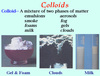38

## Solution Chemistry Solubility Define: solubility precipitate saturated solution unsaturated solution super-saturated solution

### Solubility the amount of a solute that will dissolve in a given solvent at a given temperature. Temperature is usually specified because for most solids dissolved in liquids, solubility is directly related to temperature. On the MCAT, solubility is usually measured in either g/mL, g/100mL, or mol/L. A Precipitate a solid formed inside of a solution as the result of a chemical reaction, such as the common ion effect. Precipitates only form when the ion product exceeds the solubility product constant, Ksp. For example, given the dissolution of iron(III)chloride in water [Equation: FeCl3(s)  Fe3+(aq) + 3Cl- (aq)], if NaCl is added to the solution Le Chatelier’s principle predicts that the reaction will shift to the left, reforming the solid. Saturated solution a solution that contains the maximum amount of dissolved solute it can hold. For a saturated solution the ion product equals the Ksp. Unsaturated solution any solution that contains less than its maximum amount of dissolved solute. For unsaturated solutions the Ksp is greater than the ion product. Super-saturated solution a solution that contains more dissolved solute than predicted by the solubility product constant ​in other words, the ion product exceeds the Ksp WITHOUT a precipitate forming. Supersaturated solutions usually form only when a solution is held at a higher temperature during dissolution (at which Ksp would be larger) and then slowly cooled to a temperature at which Ksp is smaller.39

41

42

## Phase ChangesGas Solubility How does solubility for a GAS dissolved in a LIQUID compare to a SOLID dissolved in a LIQUID? How does an increase & decrease in TEMPERATURE & Vp affect solubility of Gases dissolved in  liquids? Polar & Non-polar gases easily form WHAT? ​picture attached

### The solubility of gases in liquids follows a trend that isEXACTLY OPPOSITEof the solubility of solids in liquidsTemperature:  For GASES dissolved in liquids: increased temperature decreases solubility decreased temperature increases solubility Vapor Pressure, Vp Increasing the vapor pressure of gas X over a liquid:  increases the solubility of gas X in that liquid (This is why they pressurize soda pop cans with excess CO2)Polar and non-polar gases easily form: HOMOGENOUS MIXTURES (below)43

## Phase changes what 2 quantities are equal when a liquid boils?

### Atmospheric Pressure  Vapor Pressure45

46

47

48

## Phase Changes Heating Curves What does the SLOPE of the various NON-HORIZONTAL sections of the graph represent?### The SLOPE of the lines between these horizontal sections represents: THE INVERSE (ΔT/Q) of HEAT CAPACITY (Q/ΔT ​...for that particular phase of the substance Therefore,  DIFFERENT phases of the same substance usually have DIFFERENT heat capacities!as indicated by the differing slopes of those sections of the following graph (IE GAS HAS A STEEPER SLOPE)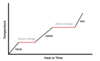49

## Phase Changes What main idea should we remember about Henry's Law?

### the solubility of a gas IN a liquid is directly proportional to the partial pressure of that gas OVER that liquid50

## Electrochemical cells Electrolytic Cells

### is essentially a galvanic cell to which an external voltage is applied  Oxidation @ anode, Reduction @ cathode Cell Potential (Eº) always NEGATIVE The sum of the externally applied voltage (Vbattery) and the negative cell potential ( -E ° cell) must be positive. Cathode = (-); Anode = (+) Note the difference compared to galvanic cells.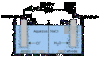52

## Gases Effusion and Diffusion (Graham's Law) Compare Effusion with Diffusion Give Graham's Law equation explain it

### Diffusion: The process by which gas molecules spread from areas of high concentration to areas of low concentration due to the random motion imparted to them as a result of their kinetic energy and collisions with other molecules.  Effusion: The diffusion of gas particles through a pin hole. A pin hole is defined as a hole smaller than the average distance a gas molecule travels between collisions.  E1/E2 = √MW2/√MW1   E1 and E2 can represent either the effusion rate or the diffusion rate of gases 1 and 2, respectively. Notice that the rate of effusion or diffusion is inversely proportional to the molecular weight of the gas.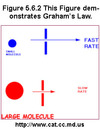53

55

56

## Phase changes Vapor Pressure  Define

### Vapor Pressure (Vp) is the partial pressure of the gaseous form of a liquid that exists over that liquid when the liquid and gas phases are in equilibrium.58

## Electrochemical cells The Galvanic (or "Voltaic") Cell Define How do they work?

### Galvanic cells convert energy into electrical energy ​By taking advantage of the difference in reduction potentials b/t two metals, a current can be spontaneously generated along a wire that connects 2 metal electrodes submerged in solutions that contain metal ions59

60

## Electrochemical cells Concentration cells How are they set up? What E° cell value do they ALWAYS have? They have a POSITIVE reduction potential,E, if there is a DIFFERENCE in the _______s of the two solutions The Nernst Equation (below) is used to calculate WHAT?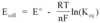### A special type of GALVANIC cell The SAME (!) electrodes and solution are used in both beakers In one beaker the metal is oxidized via its oxidation half-reaction, and in the other beaker it is reduced via its reduction half-reaction. Because the reduction potentials of oxidation and reduction half reactions for the SAME species only differ by the SIGN of E°E°cell = 0.00V It appears that nothing would happen Concentration cells are therefore NONstandard conditions by definition They have a POSITIVE reduction potential E  if: there is a difference in the MOLARITIES of the two solutions. The Nernst equation is used to calculate the cell potential based off of the E˚ of the species and the two concentrations.61

62

63

64

## Phase changes heating curves How do you calculate ΔHfusion and ΔHformation from a heating curve?  There is  NEVER _____ during a phase change?

### ΔHfusion ​The change in Q (x-axis) during the phase change from solid to liquid ΔHformation ​The change in Q (x-axis) during the phase chage from liquid to gas​ ​There is never a CHANGE IN TEMPERATURE during a phase change!!!!! The fact that heating curves are flat (i.e., horizontal, slope = 0) during the actual phase change demonstrates the following frequently-tested MCAT principle: Once a phase change starts, all of the energy goes into breaking intermolecular forces and none goes toward an increase in temperature.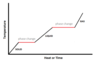65

66

67

68

## Phase Changes Vapor Pressure Raoult's Law What does Raoult's Law solve for? Give the formula for TOTAL Vapor Pressure for a Solvent mixed with a VOLATILE solute

### Raoult's Law determines the vapor pressure of a mix in which a solvent has an IMPURITY present(X=mole fraction)Total Vapor Pressure w/ a Volatile Solute = (mole fraction of solvent) X (Vp° of the solvent)+(mole fraction of the solute) X (Vp° of the solute)Vptotal =Vpsolvent + Vpsoluteaka,  (Xsolvent Vp°solvent) + (Xsolute Vp°solute)